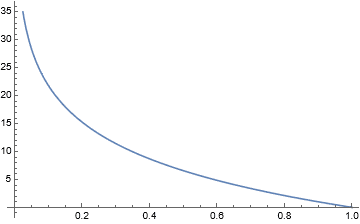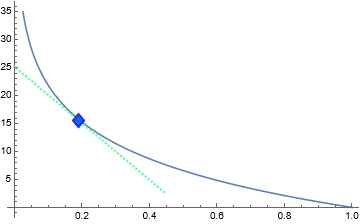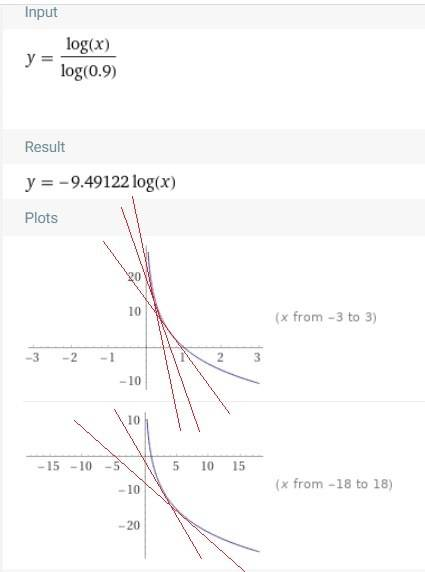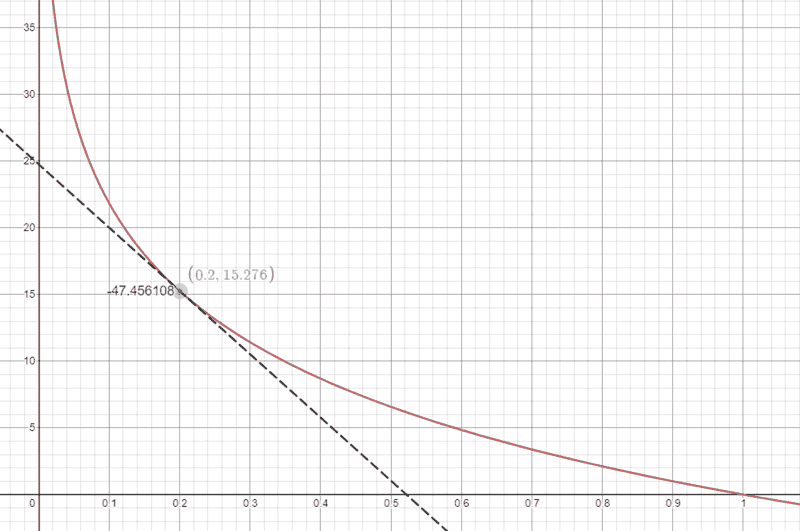# Intersection of line and curve

• B
AngleWyrm
TL;DR Summary
Trying to find coordinates of an intersection between a tangent line and a curve
I have a formula y=log(x)/log(0.9) which has this graph:I want to find the intersection of this curve and a tangent line illustrated in this rough approximation:The axes have very different scales, so the line isn't actually a slope of -1, it's just looks that way.

How can I figure out:
1). the actual slope of the line
2). the coordinates of the intersection

Gold Member
Why do don't you calculate dy/dx to get the slope of the tangential line ?

•AngleWyrm
AngleWyrm
Yeah that works; looks like about -25/0.5 = -50 slope

Homework Helper
Gold Member
How can I figure out:
1). the actual slope of the line
2). the coordinates of the intersection
If you know one of these you can determine the other, but if you don't know either then the problem is not well defined: there are an infinite number of tangent lines with some slope that intersect the curve somewhere.

Mentor
Summary:: Trying to find coordinates of an intersection between a tangent line and a curve

I want to find the intersection of this curve and a tangent line illustrated in this rough approximation:
In the lower of your two graphs, it looks like you are concerned with the point (.2, f(.2)). Is this the point of intersection for this problem? If so, that is information you should have provided.

AngleWyrm
it looks like you are concerned with the point (.2, f(.2)). Is this the point of intersection for this problem?
No, that's an estimate of the answer to the question what are the coordinates.

Mentor
No, that's an estimate of the answer to the question what are the coordinates.
Are you given the x-coordinate at the point of intersection? This is post #7, and we're still not sure exactly what you're asking.

If not, the problem is not well defined, as @pbuk said.
If you know one of these you can determine the other, but if you don't know either then the problem is not well defined: there are an infinite number of tangent lines with some slope that intersect the curve somewhere.

AngleWyrm
Yeah that works; looks like about -25/0.5 = -50 slope
If you know one of these you can determine the other
You know one of these. Carry on.

Mentor
From post #5:
it looks like you are concerned with the point (.2, f(.2)).
No, that's an estimate of the answer to the question what are the coordinates.
Is x = .2 the known x-coordinate at the point of tangency? If so, the point (.2, f(.2)) is NOT an estimate.
You know one of these.
No we don't! You haven't said what it is that is known in this problem.
I'm about to give up here and lock this thread, unless you are more forthcoming with the information of this problem.

Last edited:
Gold Member
Do you want one of these lines ?Homework Helper
Gold Member
As others have said, there are an infinite number of tangent lines.
https://www.desmos.com/calculator/ddfh2snwjy•anuttarasammyak
AngleWyrm
Is x = .2 the known x-coordinate

As others have said, there are an infinite number of tangent lines.
Are there an infinite number of tangent lines with a slope of -50?
Or does that restrict the answer set to one unique line and set of coordinates?

Last edited:
Gold Member

Are there an infinite number of tangent lines with a slope of -50?
Or does that restrict the answer set to one unique line and set of coordinates?
Say the condition is -50 slope, the solution of the equation
$$y'(x_0)=-50$$
gives unique tangential point ##(x_0,y_0)=(x_0,\frac{\log x_0}{\log 0.9})## and thus the equation of the line is
$$y-y_0=y'(x_0)(x-x_0)=-50(x-x_0)$$

•AngleWyrm
Mentor
Is x = .2 the known x-coordinate at the point of tangency?